## Example Questions

← Previous 1

### Example Question #1 : Rectangles

A rectangle has an area of 48 and a perimeter of 28.  What are its dimensions?

2 x 24

0.25 x 192

1 x 48

6 x 8

16 x 3

6 x 8

Explanation:

We can set up our data into the following two equations:

(Area) LH = 48

(Perimeter) 2L + 2H = 28

Solve the area equation for one of the two variables (here, length): L = 48 / H

Place that value for L into ever place you find L in the perimeter equation: 2(48 / H) + 2H = 28; then simplify:

96/H + 2H = 28

Multiply through by H: 96 + 2H2 = 28H

Get everything on the same side of the equals sign: 2H2 - 28H + 96 = 0

Divide out the common 2: H2 - 14H + 48 = 0

Factor: (H - 6) (H - 8) = 0

Either of these multiples can be 0, therefore, consider each one separately:

H - 6 = 0; H = 6

H - 8 = 0; H = 8

Because this is a rectangle, these two dimensions are the height and width.  If you choose 6 for the "height" the other perpendicular dimension would be 8 and vice-versa.  Therefore, the dimensions are 6 x 8.

### Example Question #2 : Rectangles

The length of a rectangle is three times its width, and the perimeter is. What is the width of the rectangle?Explanation:

For any rectangle,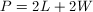, where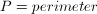,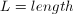, and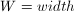In this problem, we are given that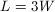(length is three times the width), so replace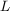in the perimeter equation with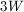: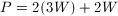Plug in our value for the perimeter,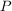: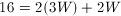Simplify: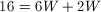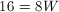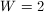### Example Question #3 : Rectangles

The area of a rectangle is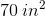. Its perimeter is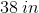. What is the length of its shorter side?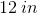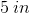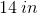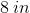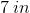Explanation:

We know that the following two equations hold for rectangles. For area: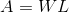For perimeter: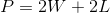Now, for our data, we know: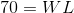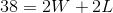Now, solve the first equation for one of the variables: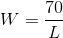Now, substitute this value into the second equation: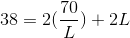Solve for: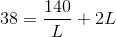Multiply both sides by: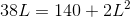Solve as a quadratic. Divide through by: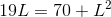Now, get the equation into standard form: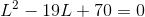Factor this: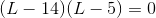This means that(or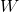) would equal eitheror. Therefore, your answer is.

### Example Question #1 : Rectangles

Given Rectangle ABCD.

Quantity A: The length of diagonal AC times the length of diagonal BD

Quantity B: The square of half of ABCD's perimeter

The two quantities are equal.

Quantity B is greater.

The relationship cannot be determined from the information given.

Quantity A is greater.

Quantity B is greater.

Explanation:

Suppose ABCD has sides a and b.

The length of one of ABCD's diagonals is given by a2+ b2 = c2, where c is one of the diagonals.

Note that both diagonals are of the same length.

Quantity A: The length of diagonal AC times the length of diagonal BD

This is c * c = c2.

Quantity A = c2 = a2+ b2

Now for Quantity B, remember that the perimeter of a rectangle with sides a and b is Perimeter = 2(a + b).

Half of Perimeter = (a + b)

Square Half of Perimeter = (a + b)2

Use FOIL: (a + b)2 = a2+ 2ab + b2

Quantity B = (a + b)2 = a2+ 2ab + b2

The question is asking us to compare a2+ b2 with a2+ 2ab + b2.

Note that as long as a and b are positive numbers (in this case a and b are dimensions of a rectangle, so they must be positive), the second quantity will be greater.

### Example Question #2 : How To Find The Length Of The Diagonal Of A Rectangle

If rectanglehas a perimeter of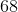, and the longer edge is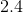times longer than the shorter edge, then how long is the diagonal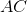?Explanation:

Lets call our longer side L and our shorter side W.

If the perimeter is equal to 68, then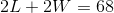.

We also have that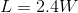.

If we then plug this into our equation for perimeter, we get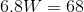.

Therefore,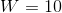and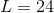. Using the Pythagorean Theorem, we have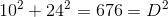so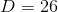.

### Example Question #1 : How To Find The Area Of A Rectangle

If a rectangle's width is increased by 20%, and its length is decreased by 20%, which statement most accurately reflects the rectangle's change in area?

The rectangle's area will be increased by 10%.

The rectangle's area will be reduced by 4%.

The rectangle's area will not change.

The rectangle's area will be increased by 4%.

The rectangle's area will be decreased by 10.%

The rectangle's area will be reduced by 4%.

Explanation:

If = A, then we will call the new area B. B results when has been decreased by 20% and has been increased by 20%: in other words, 80% of l and 120% of w.

This is expressed as (0.8) l * (1.2) w = B

The simplest way to determine the result is to create rectangles with actual integers and see what happens. Let's say our first rectangle is a square 10 units by 10 units.

10 * 10 = 100 for our A.

Plugging these numbers into our new equation gives us:

(0.8)10 * (1.2)10 = 96.

So the final step is to compare the areas.  Because we used a 10 x 10 rectangle as our example, this step will be easy, since 100% = 100.

96/100 = 0.96

0.96 * 100 = 96%

Which reflects a 4% reduction in the size of the rectangle.

Any other length and width used for this rectangle will result in a 4% reduction in size when the above parameters are applied.

### Example Question #5 : Rectangles

Amy is building a rectangular patio in her backyard. She plans on making the patio 8 feet wide by 12 feet long. The rectangular bricks she is using are 6 inches long and 4 inches wide. How many bricks will Amy need to build her patio?

384

96

576

424

782

576

Explanation:

Because Amy is building her patio in a rectangle, and the bricks are rectangular, the simplest way to find this solution is to determine how many bricks long and how many bricks wide her patio will be.

To do this, begin by converting the patio's dimensions into inches, since the bricks Amy is using are measured in inches.

Length:

12 feet * 12 inches in a foot = 144 inches long.

Width:

8 feet * 12 inches in a foot = 96 inches wide.

Now, all that's left to do is figure out how many bricks will fit in these spaces.  Let's be intuitive and assume the long sides of the bricks will align with the long sides of the patio.

144 inches long / bricks that are 6 inches long = 24 bricks long

Amy will need columns of 24 bricks to cover the length of her patio.  Now, for the width.

96 inches wide / bricks that are 4 inches wide = 24 bricks wide

Amy will need rows of 24 bricks to cover the width of her patio.

To determine how many bricks Amy will need in total, the last step is to multiply how many rows of bricks she will need by how many columns.

24 bricks to a row * 24 bricks to a column = 576

Amy needs 576 bricks to build her patio.

### Example Question #1 : Rectangles

Quantity A: The area of a rectangle with a perimeter of 40

Quantity B: The area of a triangle with a perimeter of 40

The two quantities are equal

The relationship cannot be determined from the information given

Quantity B is greater

Quantity A is greater

The relationship cannot be determined from the information given

Explanation:

The area of the two shapes depends on their dimensions, which can vary greatly among different combinations. A rectangle with a perimeter of 40 could have dimensions of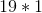(smaller area) or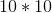(larger area), for instance. Similarly, a triangle could have sides of 10--11--19 (smaller area) or 13--13--14 (larger area). Thus, the relationship cannot be determined without more specific details.

### Example Question #7 : Rectangles

The perimeter of a rectangle is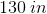. One side is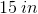. What is the area of this rectangle?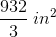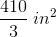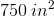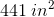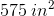Explanation:

Recall that perimeter is defined as:For our data, this is: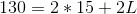Solve for: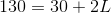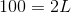Therefore,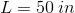This means that the area of the rectangle is equal to: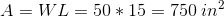### Example Question #8 : Rectangles

Rectangle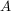has a length of 20 inches and a width of 3 inches. Rectanglehas a length of 9 inches and a width of 10 inches. By what number must the area of rectanglebe multiplied by to equal the area of rectangle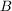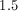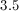Explanation:

Area of rectangleis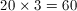.

Area of rectangleis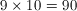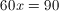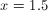← Previous 1

Tired of practice problems?

Try live online GRE prep today.